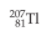×
Get Full Access to Introductory Chemistry - 5 Edition - Chapter 17 - Problem 53p
Get Full Access to Introductory Chemistry - 5 Edition - Chapter 17 - Problem 53p

×

# How many protons and neutrons are in this nuclide?ISBN: 9780321910295 34

## Solution for problem 53P Chapter 17

Introductory Chemistry | 5th Edition

• Textbook Solutions
• 2901 Step-by-step solutions solved by professors and subject experts
• Get 24/7 help from StudySoup virtual teaching assistantsIntroductory Chemistry | 5th Edition

4 5 1 312 Reviews
17
2
Problem 53P

How many protons and neutrons are in this nuclide?Step-by-Step Solution:
Step 1 of 3This nucleus has 81 number of protons

Number of neutrons =207-81

=126 neutrons .

#### Explanation:

Two numbers are used to identify a nucleus:

Atomic number -- number of just protons in the nucleus. The atomic number determines what an element is chemically, for the number of protons determines the number of electrons needed to balance the charges in an atom, which in turn determines the electron configuration the atom will adopt.

Mass number is the number of protons plus neutrons. This is roughly proportional to the total mass of the atom.

Mass number is also a key property of atoms because we need to know the masses of atoms to understand how a given amount of material we weigh out will react .

That's why chemists commonly identify an isotope with the element, which implies its atomic number, and then the mass number.

Step 2 of 3

Step 3 of 3

##### ISBN: 9780321910295

Unlock Textbook Solution